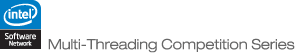JOINProblem Statement

Problem: NearestNeighbors

### Problem Statement

You will be given two sets of points in N-dimensional space: A and B. Your task is: for every point b in set B, find the point a in set A that is closest to b (using Euclidean distance).

More specifically, you will be given String[]'s A and B, where each element represents a point. Each element is a single-space delimited list of N reals between 0 and 1 (inclusive), where the ith real corresponds to the ith dimension. You should return a int[] with |B| elements. Element j of the return should be the index into A of the point closest to the jth element of B (all indexed from 0).

Scoring will be based on the quality of your algorithm's return. For a test case, your score will be proptional to |B|*N/sum(distance2). Your final score will be the sum of your scores on the individual test cases.

The test cases will be generated as follows:
1. The number of elements in A and B and the number of dimensions are chosen uniformly in the given ranges.
2. A random integer G is chosen uniformly between 1 and 100.
3. G Gaussian distributions are created. For each distribution, a mean and standard deviation are chosen randomly at uniform for each dimension between 0 and 1.
4. A probability p is generated uniformly between 0 and 1
5. Next, the points of A and B are generated. Each point either comes from one of the Gaussian distributions (with probability p), or is chosen uniformly at random from the unit hyper-cube. If a point is generated from one of the gaussian distributions, but falls outside the unit hyper-cube, that point is regenerated (from the same distribution) until it falls within the unit hyper-cube. Points generated from the Gaussian distributions have each of their dimensions generated independently from a normal curve according to the mean and standard deviation for that dimension.

### Definition

 Class: NearestNeighbors Method: nearest Parameters: String[], String[] Returns: int[] Method signature: int[] nearest(String[] A, String[] B) (be sure your method is public)

### Notes

-The time limit is 15 seconds.
-The memory limit is 1024 megabytes.
-The thread limit is 32 threads (including the primary thread).
-An invalid return will receive a score of 0.

### Constraints

-A and B will each contain between 1 and 50,000 elements, inclusive.
-N will be between 10 and 50, inclusive.
-Each decimal number will have exactly 6 digits after the decimal point.

### Examples

0)

`In this test case, A has 10 elements, while B has 10 elements.  There are 15 dimensions.`
1)

`In this test case, A has 20 elements, while B has 20 elements.  There are 39 dimensions.`
2)

`In this test case, A has 50 elements, while B has 50 elements.  There are 48 dimensions.`
3)

`In this test case, A has 100 elements, while B has 100 elements.  There are 50 dimensions.`
4)

`In this test case, A has 21752 elements, while B has 45585 elements.  There are 30 dimensions.`
5)

`In this test case, A has 39555 elements, while B has 23042 elements.  There are 32 dimensions.`
6)

`In this test case, A has 49232 elements, while B has 49679 elements.  There are 19 dimensions.`
7)

`In this test case, A has 42095 elements, while B has 35872 elements.  There are 49 dimensions.`
`In this test case, A has 18979 elements, while B has 36506 elements.  There are 16 dimensions.`
`In this test case, A has 10555 elements, while B has 45064 elements.  There are 47 dimensions.`